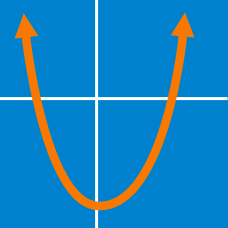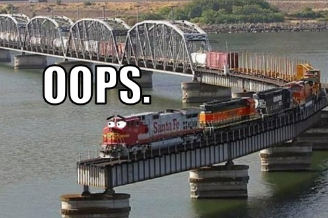Algebra

Quadratic Equations: Level 3 ChallengesYou went on a 360km train ride but you are late! If the train had just been 5 km/hr faster, then the train ride would have been one hour shorter.

How fast was the train going?

If $a$ and $b$ are non-zero real numbers, simplify: $\left(a + \frac1a\right)^2+\left(b + \frac1b\right)^2 + \left(ab + \frac1{ab}\right)^2 \\ - \left(a + \frac1a\right)\left(b + \frac1b\right)\left(ab + \frac1{ab}\right)$

$x(x+1)(x+2)(x+3)=120$

Find the sum of all the real roots of the equation above.

$\large (x^2 - 5x + 5)^{(x^2 - 11x + 30)} = 1$

How many different integers satisfy the equation above?

How many real value(s) of $x$ exist satisfy the equation $\left( 5+2\sqrt{6} \right)^{x^2-3}+\left( 5-2\sqrt{6} \right)^{x^2-3}=10 ?$

×